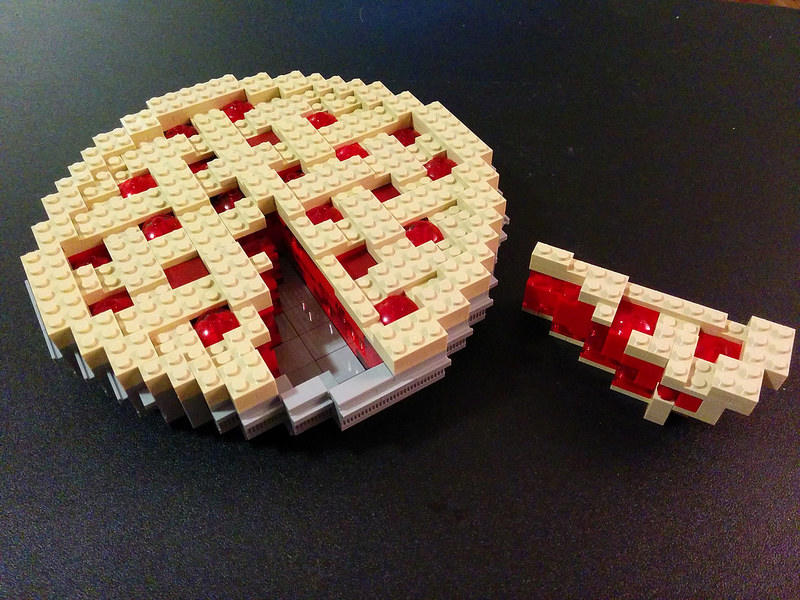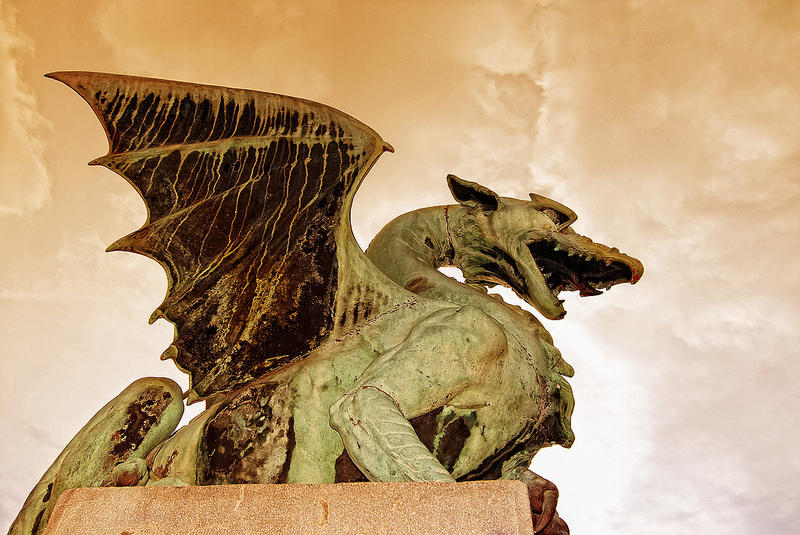Mathematics is defined as “The abstract science of number, quantity, and space, either as abstract concepts (pure mathematics), or as applied to other disciplines such as physics and engineering (applied mathematics).”

Confusing, right?

For years pupils have seen maths as merely a set of ideas such as:

• Geometry,
• Calculation, mental maths
• Pythagoras’ or Thales' theorem,
• Algebra, arithmetic and trigonometry,
• Multiplication tables, division, fractions etc.

But maths is much more than just multiplication and division! It's a true scientific discipline full of all sorts of secrets and mysteries that exist outside maths class.

Since antiquity, mathematicians and researchers have been fascinated by the possibilities and peculiarities of their discoveries. Fascinating figures, absurd paradoxes, riddles... Could this tempt you to see Mathematics homework differently?The best Maths tutors available5 (19 reviews)
Andrew
RM30
/h1st lesson free!5 (14 reviews)
Yun hang
RM40
/h1st lesson free!5 (6 reviews)
Abdul muhaimin
RM35
/h1st lesson free!5 (11 reviews)
Kai lin
RM25
/h1st lesson free!5 (3 reviews)
Yutesh
RM40
/h1st lesson free!4.9 (10 reviews)
Felicia luvena
RM35
/h1st lesson free!Syed abdul jabbar
RM25
/h1st lesson free!4.9 (6 reviews)
Suganisha
RM30
/h1st lesson free!5 (19 reviews)
Andrew
RM30
/h1st lesson free!5 (14 reviews)
Yun hang
RM40
/h1st lesson free!5 (6 reviews)
Abdul muhaimin
RM35
/h1st lesson free!5 (11 reviews)
Kai lin
RM25
/h1st lesson free!5 (3 reviews)
Yutesh
RM40
/h1st lesson free!4.9 (10 reviews)
Felicia luvena
RM35
/h1st lesson free!Syed abdul jabbar
RM25
/h1st lesson free!4.9 (6 reviews)
Suganisha
RM30
/h1st lesson free!

## Pi and its Legacy as the Most Beautiful Formula in Mathematics

When you learn maths it is impossible to ignore Pi.

Today Pi is valued approximately at 3.14 and it is this very number that has fascinated and intrigued mathematicians and scientists since antiquity!Pi, a mathematical constant that has its own official day, 14th of March! A day to celebrate the fun maths facts of Pi. Source: visualhunt

In mathematics Pi is used to calculate the volume of a sphere or the ratio of a circle's circumference to its diameter.

The discovery of this number dates back to antiquity, when scholars and mathematicians studied the number Pi to determine the most representative value possible.

However, it wasn’t until Archimedes and his essay "The measurement of a circle" that we arrived at the approximation we know today: 220/71 <Pi <22/7.

More than 2,000 years after Archimedes’ discovery, the mathematical formula is still being used.

Even if computers are now able to calculate Pi to several thousand decimal places, the number remains a mystery. A myth in the world of maths, science and technology.

Why the fascination with Pi?

Nearly four thousand years after its discovery, the value of Pi has become a cornerstone in basic mathematics. A mathematical formula that is taught to kids from a very young age: from Secondary school to Higher Education and beyond.

A number with many secrets and mysterious properties:

• Pi is irrational: it is impossible to write it in fraction form because of its infinite decimal places...
• Pi is transcendent: mathematically speaking, the value of Pi cannot be the solution to any equation. But theoretically, Pi is directly linked to several mathematical constants, such as the Fibonacci sequence.
• Pi is approximate: since it is impossible to define the exact value of Pi, its value remains approximate but, paradoxically, it allows us to make extremely accurate calculations.
• Pi is everywhere: in addition to its ubiquity in geometry, Pi is widely used in statistics and probability.

Its ubiquitous presence throughout the world and in science makes Pi one of the greatest mathematical constants for researchers and maths enthusiasts! It is found everywhere, in trigonometry, geometry, physics, chemistry, biology, and so on.

## The Most Useful and Famous Mathematical Paradoxes

By definition a paradox (in the broad sense) is an absurdity, a false conclusion that stems from correct reasoning.

Example: “One Law says: “It is forbidden to forbid”. The content of the law contradicts the law itself. It would therefore be forbidden to say that it is forbidden to forbid and therefore forbidden to say that it is forbidden to say that it is forbidden to forbid.”

Whether in trigonometry, arithmetic or probability, many mathematical paradoxes have become famous, here are a few.

### The False Paradox of Achilles and the Tortoise

Surely one of the most famous mathematical paradoxes comes from the Greek Philosopher, Zeno of Elea (430 BC).

His theory , in light of the mathematical knowledge of the time, was that if the tortoise was given a head start in a race against Achilles, Achilles could never catch up to the tortoise because every time he did, the tortoise would open a new gap between them.

Even though this assertion seems completely ludicrous it proves devilishly difficult to explain.

### The Paradox of the Missing Square

Let's remain in the realm of the absurd, but this time with geometry. The missing square is a logical mathematical hypothesis, that ultimately it rests only on a visual illusion, thus leading to a conclusion that is obviously false.

The problem: based on the tangram model, it is a question of reconstructing a triangle (already formed) with other geometric shapes (squares, rectangles, triangles, etc.).

The answer puts a small square of vacuum at the center of the triangle once reconstitution is complete. So, there would be, during the reconstruction, a loss of area...

The answer to the puzzle: it is obviously impossible! Neither triangle is a "true" triangle because of the slight curve in the hypotenuse, despite how it looks to the human eye. The small empty space is in fact only the result of a small deformation of the perfect triangle with its slightly rounded edges.

These paradoxes are highly valued by mathematics teachers who like to use maths puzzles and games for kids to make students open their eyes to new ideas in maths (complex theorems, equations, geometric figures, etc.)

Today there is no need to have a Mathematics degree or to be an engineer in science and technology to understand the absurdity of these paradoxes. But, at the time, they certainly divided common opinion!

It is the study of these assertions that, years later, enabled the development of theorems, mathematical exercises, equations and mathematical notions to refute these hypotheses.

During your next maths class do not hesitate to ask your teacher if he/she knows any other wacky mathematical paradoxes. Among other topics discussed, for example:

• literal calculation,
• using a scientific calculator,
• probability,
• symmetry,
• Equation (subtraction, addition, division, multiplication),
• Polygon, polynomial,
• Relative numbers...

## The Greatest Mathematical Mysteries

Beyond your classic times tables, mental arithmetic, calculus, boring maths lessons (not my words...) and endless equations, math can actually become a captivating science to study!

When you take a closer look at maths, you can easily come across a number of unsolved riddles and problems. The fascination with whole numbers, rather than strange mathematical constants, and so on.

One of the biggest mathematical mysteries lies in the endless debate: Did man invent or discover mathematics?

Since antiquity, mathematicians and researchers have been trying to answer this question.

By using algebra, literal calculation and counting, man has managed to explore the world and the nature that surrounds it.

Fascinating documentaries retrace the history of mathematics and highlight the discoveries of the greatest names in this science: Pythagoras, Galileo, Newton and, of course, Einstein.

Mathematical mysteries and enigmas that will surely never fail to baffle us!

### The 7 Most Puzzling Mathematical Riddles of the Millennium

Seven maths exercises have been identified by researchers as the most challenging!

Hypotheses, conjectures, number sequences  equations, all types of maths problems that, in 2016, have never been solved!

Solving these problems requires a very advanced level of applied mathematics, only a handful of mathematicians and researchers have the opportunity to tackle them. We are certainly very far from secondary school algebra lessons...

The icing on the cake: the Clay Institute of Mathematics is offering \$1 million for each problem solved.

Doing maths can even make you rich! Very rich in fact! That certainly makes mathematics homework more appealing, don’t you think? So why not get some maths help to get you started on the right track!

### Mysterious Numbers...

Who says math is just a bunch of numbers?

Like Pi which we discussed at the beginning of this article, researchers and maths enthusiasts have long been fascinated by numbers.

Between scientific facts and myths, let's try to understand these mysterious numbers by looking at the following as examples:

• The Golden ratio (Phi): According to mathematicians, the number Phi could be present in all things in the universe, in human beings, animals, plants, spaces, etc. The factor of the proportions of our universe. Its value (about 1,618) could allow, by associating it with the Fibonacci series, to draw shapes with perfect proportions.
• Leonard De Vinci, through his work “The Vitruvian Man”, was the first to declare that all the parts of the human body have the value Phi as a common denominator...
• The number 7: present in all of our cultures, the number 7 remains a mystery for mathematicians. The 7 wonders of the world, 7 days of the week, 7 league boots...A ubiquitous figure in cultures, religions, and even maths! (7 acute triangles divide an obtuse triangle, 7 is a magic number, a prime number, 7 models of disaster in mathematics, etc.).

Unsettling, isn't it?

## How to Use Maths to Work Out Who is the Main Character in GOT

What's the link between Game of Thrones and Mathematics

At first glance, there isn't one. Except when two maths teachers decide, thanks to their subject, to mathematically calculate who is the main character in this series with great results.

This exercise becomes all the more challenging when you think about the number of characters in this series based on the novels of R.R Martin.

To solve the problem the two researchers, named Beveridge and Shan, did not use typical theorems or arithmetic, but a new and growing science coupled with a classic mathematical formula.

They used the science of networks: a scientific discipline which deals with the relationships, links and interconnections between things. In this case, the interactions between the most recurring characters of the Iron Throne!I'm starting a petition to include GOT maths games in the maths curriculum across schools nationwide! Who’s with me? Source: visualhunt

A new science that can also be applied in physics or biology.

Using 6 different centralities and scales, as well as Google's PageRank algorithm, the researchers were able to group the characters into 7 different communities. With the theory of graphs, they then modelled their result with vertices and edges.

For once we are able to say that maths and science is everywhere! At the centre of our culture, our universe...

A school subject full of treasures, enigmas, and mysteries. A great way to arouse students' curiosity, don't you think?

Younger kids can also be introduced to mathematics and its ideas, through various fun activities such as maths games, logic games and magic calculations, for example.

From primary school to A-levels, the Board of Education could easily propose changes to the curriculm to make maths classes way more fun and help kids learn math online faster!

Tackling science and maths using popular culture and fun games (see Games Of Thrones) would definitely help students learn. There's tons of free maths games online, ask your maths teacher or tutor if you can play maths puzzles in class! Once you open up these new possibilities, students will start to enjoy problem solving and discover the hidden treasures of this subject!

Maths and engineering schools better watch out!The platform that connects tutors and students5.00 (1 rating(s))Loading...